## The Topology of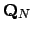(is Weird)

Definition 16.2.10 (Connected)   Let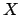be a topological space. A subset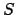ofis if there exist open subsets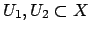with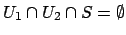and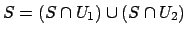with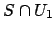and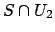nonempty. Ifis not disconnected it is .

The topology onis induced by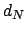, so every open set is a union of open balls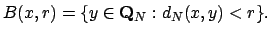Recall Proposition 16.2.8, which asserts that for all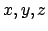,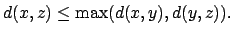This translates into the following shocking and bizarre lemma:

Lemma 16.2.11   Suppose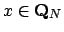and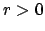. If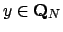and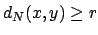, then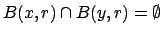.

Proof. Suppose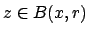and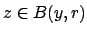. Then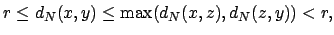a contradiction.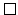You should draw a picture to illustrates Lemma 16.2.11.

Lemma 16.2.12   The open ball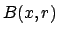is also closed.

Proof. Suppose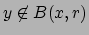. Then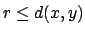so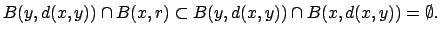Thus the complement ofis a union of open balls.The lemmas imply thatis , in the following sense.

Proposition 16.2.13   The only connected subsets ofare the singleton sets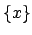forand the empty set.

Proof. Suppose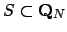is a nonempty connected set and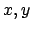are distinct elements of. Let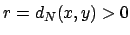. Let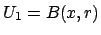and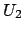be the complement of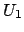, which is open by Lemma 16.2.12. Thenandsatisfies the conditions of Definition 16.2.10, sois not connected, a contradiction.William Stein 2004-05-06